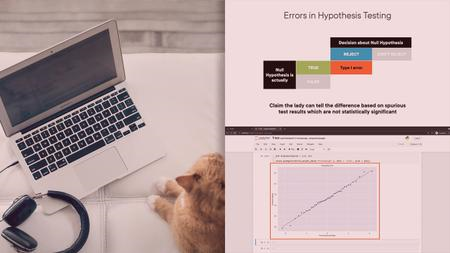﻿ Foundations of Statistics and Probability for Machine Learning » GFXhome WS

Information of news
16-11-2021, 07:34

### Foundations of Statistics and Probability for Machine Learning

Category: TutorialsDuration: 2h 12m | Video: .MP4, 1280x720 30 fps | Audio: AAC, 48 kHz, 2ch | Size: 217 MB
Level: Beginner | Genre: eLearning | Language: English

This course will teach you the concepts, theory, and implementation of basic statistics, probability, hypothesis testing, and regression analysis required to build and interpret meaningful machine learning models.
Learning the importance of p-values and test statistics and how these can be used to accept or reject the null hypothesis can lead you to explore the different types of t-tests and learn to choose the right one for your use case.
In this course, Foundations of Statistics and Probability for Machine Learning, you will learn to leverage statistics for exploratory data analysis and hypothesis testing.
First, you will explore measures of central tendency and dispersion including mean, mode, median, range, and standard deviation.
Then, you will explore the basics of probability and probability distributions and learn how skewness and kurtosis can give you important insights into your data.
Next, you will discover how you can perform hypothesis testing and interpret the results of these statistical tests.
Finally, you will learn how to perform and interpret regression models both simple regression with a single predictor and multiple regression with multiple predictors, and you will evaluate your regression models using R-squared and adjusted R-squared and understand the t-statistic and p-value associated with regression coefficients.
When you are finished with this course, you will have the skills and knowledge of statistics and data analysis needed to effectively explore and interpret your data as a precursor to applying machine learning techniques.Site BBcode/HTML Code:
Dear visitor, you went to the site as unregistered user.